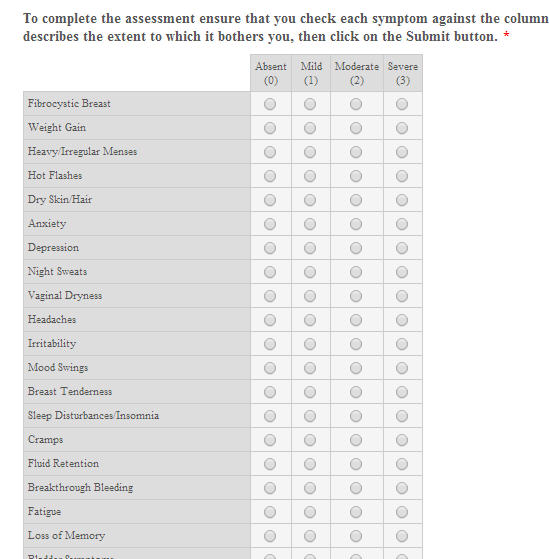# Can I use a calculation on a matrix?

•Asked on August 22, 2014 at 02:12 PM

I want to put a menopause self assessment on my web site. I created a form using Jotform that contains a matrix with radio buttons. My columns (options) are:

- Absent

- Mild

- Moderate

- Severe

I would like to be able to assign each option a value (0 - 3) and calculate the total (this would give an indication of severity of symptoms).

Is this possible to do with Jotform?

Thank you,

Stephanie•jonathan
Answered on August 22, 2014 at 03:56 PM

Hi Stephanie,

Unfortunately you cannot use the Matrix table field for calculations because it lacks the Calculation Values property that other fields have that is needed for calculation.What you can try using instead is he regular Radio buttons(found in Form Tools) as it has the Calculation Values property.Here is a test form http://www.jotform.me/form/42336401771449  you can try.

In the test form I have setup the Calculation Widget with calculation formula like this.So every time an option is selected on each of the Radio items, the grand Total is calculatedCheck also this related user guide

http://www.jotform.com/help/259-How-to-perform-calculation-in-the-form

Hope this help. Inform us if you need more assistance.

Thanks!

•Answered on June 01, 2015 at 03:00 PM

Hi there,

Is the answer to this question still the same? i.e. Is it still impossible to create a matrix with calculation values?
•Mike_G
Answered on June 01, 2015 at 05:21 PM